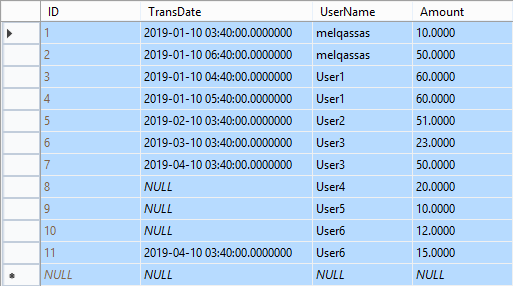# Get the last record added per day group by the user in SQLIn this post, I am gonna explain

How to get the last record added per day for each user in SQL.

## Get the last entry added per day group by the user in SQL

Consider you have a [Transaction] table to store multiple transactions for different users daily!

The [Transaction] table structure is simple like the below one:

• ID.
• Transaction Date.
• Amount…etc.

You may be also interested to read Get the first record added per day group by the user in SQL.

In this table, the user add multiple transactions per day! and you would like to know what’s the last record added per day for each user!

Actually, there are multiple way to query the last transaction added for each user per day in SQL.

In this post, I am gonna to explore two different queries as the following:

### Using row_number() function

Firstly, Let’s explain what’s the row_number() function?

• It’s a function that assigns a sequential integer to each row within a partition of a result set.
• You can use it with “PARTITION BY” to divide the result set into partitions per specific column, actually, it’s an optional part, if you don’t use it, the function will treat all rows of the query result set as a single group.

#### Steps

1. Get all rows from [Transaction] table with a sequential row number for each “[UserName]” ordered by “[Transaction Date]” Descending.
2. Use the “WITH” clause to give the above query a block name (MaxTransTable).
3. Get all records filtered by the sequential number ID= 1 and the [Transaction date] is not null.
##### Get all rows from [Transaction] table

Get all rows from [Transaction] table with a sequential row number for each “[UserName]” ordered by “[Transaction Date]” Descending.

```SELECT row_number() over(PARTITION BY UserName ;
ORDER BY TransDate DESC) AS ID,
[TransDate],
[Amount]
FROM [dbo].[Transactions] )```
##### Use the “WITH” clause

Use “WITH” clause to give the above query a block name (MaxTransTable)

```WITH MaxTransTable AS
ORDER BY TransDate DESC) AS ID,
[TransDate],
[Amount]
FROM [dbo]. [Transactions])```
##### Get all filtered records

Get all records filtered by the sequential number ID= 1 and the [Transaction date] is not null.

```WITH MaxTransTable AS
ORDER BY TransDate desc) AS ID,
[TransDate],
[Amount]
FROM [dbo]. [Transactions])
SELECT *
FROM MaxTransTable
WHERE MaxTransTable.ID = 1
AND MaxTransTable.[TransDate] IS NOT NULL```

The final result would be as the following:

You may be also interested to read SQL Server: Get the Detailed Information Via SERVERPROPERTY.

### Using Inner Join

It’s another query that you can use to get the last entry added for a specific user per day.

#### Steps

1. Get all required field from the main table [Transaction].
2. Get all records aggregated with Maximum function on the [TransDate] grouped by [UserName] field.
3. Use “WITH” clause to give the above query a block name (MaxTransTable).
4. Perform inner join between main [Transaction] table and [MaxTransTable].
##### Get all required field

Get all required field from the main table [Transaction].

```SELECT [Transactions].UserName,
[Transactions].TransDate,
[Transactions].Amount
FROM [dbo].[Transactions]```
##### Get all records aggregated

Get all records aggregated with Maximum function on the [TransDate] grouped by [UserName] field.

```SELECT [UserName],
Max([TransDate]) AS MaxDate
FROM [dbo].[Transactions]
##### Use “WITH” clause
```WITH MaxTranstable AS
max([TransDate]) AS MaxDate
FROM [dbo]. [Transactions]
##### Perform inner join

Perform inner join between main [Transaction] table and [MaxTransTable].

Use “WITH” clause to give the above query a block name (MaxTransTable).

```WITH MaxTranstable AS
max([TransDate]) AS MaxDate
FROM [dbo]. [Transactions]
[Transactions].TransDate,
[Transactions].Amount
FROM [dbo].[Transactions]
AND [Transactions].TransDate = MaxTranstable.MaxDate```

The final result would be like

#### Which Query is the best one?

Although the two queries generate the same result, but as per my experiment using actual execution plan, the row_number() query is faster than the inner join query!

###### Applies To

SQL Server 2012 and later.

###### Conclusion

In this post, I have explored two queries to get the last entry added per day for each user in SQL.

1.